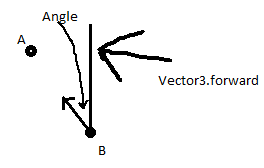# How do i get the angle of two Vector 3's?

I’m still fairly new to Unity and C#, so this probably looks a bit sloppy. I’m working on a script that’s supposed to log the angle to Vector3 (Player’s position) from Vector3 b (Objects position), and I’m using Vector3.Angle, but it’s not working quite like I want it to, as the angle only goes up to about 76 and then starts going back down again, and if I walk further from the object the angle shouldn’t change because I don’t want the distance between them to affect it, but it does for some reason.

Thanks in advance for any help.

Here’s my code so far:

``````	public Vector3 PlaPoint;
public Vector3 BombPoint;

public Transform PlayerPos;
public Transform BombPos;

public float angle;

void OnTriggerStay () {

Vector3 BombPoint = new Vector3 (BombPos.position.x, 0, BombPos.position.z);
Vector3 PlaPoint = new Vector3 (PlayerPos.position.x, 0, PlayerPos.position.z);

angle = Vector3.Angle (BombPoint, PlaPoint);

Debug.DrawLine (PlaPoint, BombPoint);
Debug.Log ("Angle : " + angle);
}
``````

By the way the Object in question is supposed to be a bomb, thus it being called BombPos and BombPoint.

You can’t get the angle between points without a reference to something else. Vector3.Angle is used to get the angle between two directions.So if you look at this image, we can get the angle as indicated by doing `Vector3.Angle(a-b, Vector3.forward)`.

Without more information on exactly what angle you are trying to get (and in reference to what), I can’t tell you exactly what you will need to pass in to Vector3.Angle. But regardless, it will need to be directions, not positions.